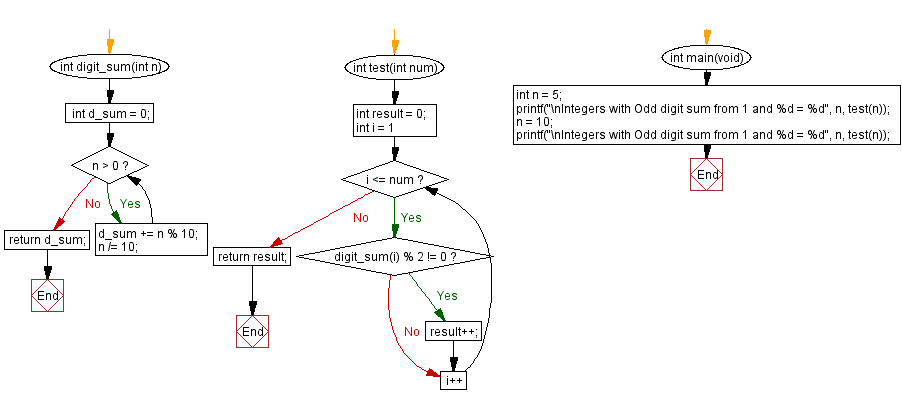﻿ C - Count Integers with Odd digit sum

# C Programming: Count Integers with Odd digit sum

## C Programming Mathematics: Exercise-38 with Solution

Accept a positive integer (n) from the user. Write a C program that counts the number of positive integers from 1 to n whose digit sums are odd.

Example:
Input: n = 5
Integers less than or equal to 5 whose digit sums are odd are 1,3 and 5.
Output: 3
Input: n = 10
Integers less than or equal to 5 whose digit sums are odd are 1, 3, 5, 7, 9 and 10 (1+0 =1)
Output: 6

Test Data:
(5) -> 3
(10) -> 6
(11) -> 6

Sample Solution:

C Code:

``````#include <stdio.h>

int digit_sum(int n) {
int d_sum = 0;
while (n > 0) {
d_sum += n % 10;
n /= 10;
}
return d_sum;
}

int test(int num) {
int result = 0;
for (int i = 1; i <= num; i++) {
if (digit_sum(i) % 2 != 0) {
result++;
}
}
return result;
}
int main(void) {
int n = 5;
printf("\nIntegers with Odd digit sum from 1 and %d = %d", n, test(n));
n = 10;
printf("\nIntegers with Odd digit sum from 1 and %d = %d", n, test(n));
}
```
```

Sample Output:

```Integers with Odd digit sum from 1 and 5 = 3
Integers with Odd digit sum from 1 and 10 = 6
```

Flowchart:C Programming Code Editor:

Improve this sample solution and post your code through Disqus.

What is the difficulty level of this exercise?

Test your Programming skills with w3resource's quiz.

﻿